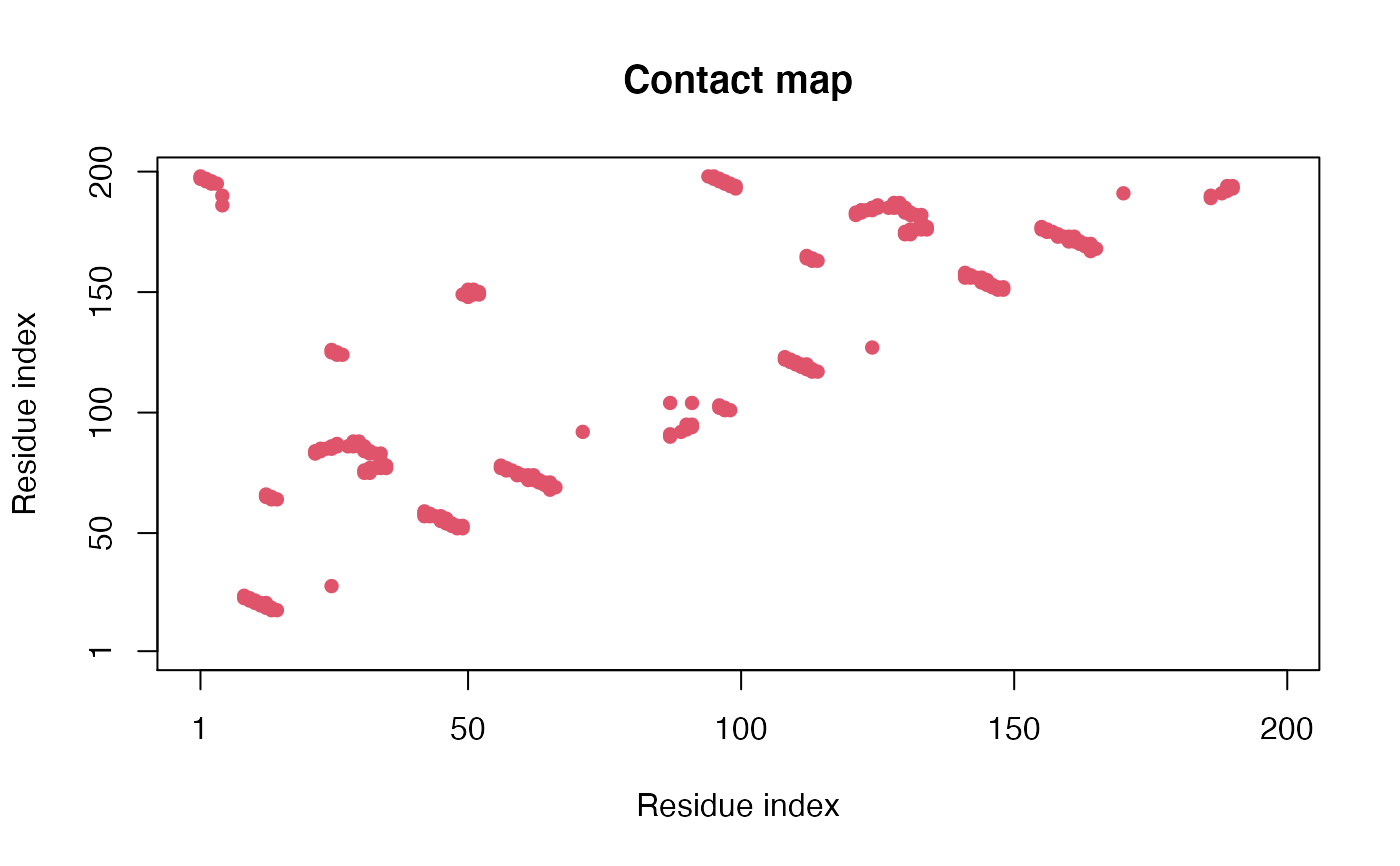Construct a Contact Map for Given Protein Structure(s).

cmap(...)

# S3 method for default
cmap(...)

# S3 method for xyz
cmap(xyz, grpby = NULL, dcut = 4, scut = 3, pcut=1, binary=TRUE,
mask.lower = TRUE, collapse=TRUE, gc.first=FALSE, ncore=1, nseg.scale=1, ...)

# S3 method for pdb
cmap(pdb, inds = NULL, verbose = FALSE, ...)

# S3 method for pdbs
cmap(pdbs, rm.gaps=FALSE, all.atom=FALSE, ...)

## Arguments

xyz numeric vector of xyz coordinates or a numeric matrix of coordinates with a row per structure/frame. a vector counting connective duplicated elements that indicate the elements of xyz that should be considered as a group (e.g. atoms from a particular residue). a cutoff distance value below which atoms are considered in contact. a cutoff neighbour value which has the effect of excluding atoms that are sequentially within this value. a cutoff probability of structures/frames showing a contact, above which atoms are considered in contact with respect to the ensemble. Ignored if binary=FALSE. logical, if FALSE the raw matrix containing fraction of frames that two residues are in contact is returned. logical, if TRUE the lower matrix elements (i.e. those below the diagonal) are returned as NA. logical, if FALSE an array of contact maps for all frames is returned. logical, if TRUE will call gc() first before calculation of distance matrix. This is to solve the memory overload problem when ncore > 1 and xyz has many rows, with a bit sacrifice on speed. number of CPU cores used to do the calculation. ncore>1 requires package ‘parallel’ installed. split input data into specified number of segments prior to running multiple core calculation. See fit.xyz. a structure object of class "pdb", obtained from read.pdb. a list object of ATOM and XYZ indices as obtained from atom.select. logical, if TRUE details of the selection are printed. a ‘pdbs’ object as returned by read.fasta.pdb, read.all, or pdbaln. logical, if TRUE gapped positions are removed in the returned value. logical, if TRUE all-atom coordinates from read.all are used. arguments passed to and from functions.

## Details

A contact map is a simplified distance matrix. See the distance matrix function dm for further details.

Function "cmap.pdb" is a wrapper for "cmap.xyz" which selects all ‘notwater’ atoms and calculates the contact matrix grouped by residue number.

## Value

Returns a N by N numeric matrix composed of zeros and ones, where one indicates a contact between selected atoms.

Grant, B.J. et al. (2006) Bioinformatics 22, 2695--2696.

## Author

Barry Grant

dm, dccm, dist, dist.xyz

## Examples


pdb <- read.pdb( system.file("examples/hivp.pdb", package="bio3d") )

## Atom Selection indices
inds <- atom.select(pdb, "calpha")

## Reference contact map
ref.cont <- cmap( pdb$xyz[inds$xyz], dcut=6, scut=3 )
plot.cmap(ref.cont)if (FALSE) {
trj <- read.dcd( system.file("examples/hivp.dcd", package="bio3d") )
## For each frame of trajectory
sum.cont <- NULL
for(i in 1:nrow(trj)) {

## Contact map for frame 'i'
cont <- cmap(trj[i,inds\$xyz], dcut=6, scut=3)

## Product with reference
prod.cont <- ref.cont * cont
sum.cont <- c(sum.cont, sum(prod.cont,na.rm=TRUE))
}

plot(sum.cont, typ="l")
}